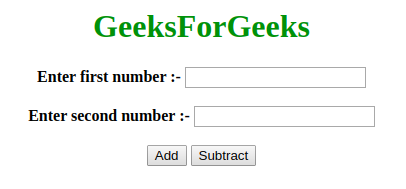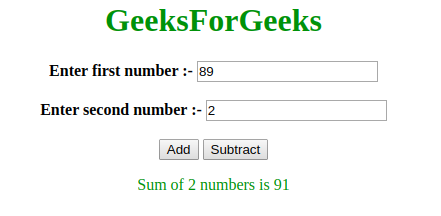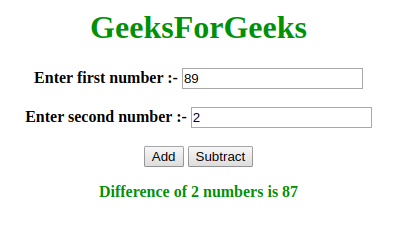Related Articles

# How to change the value of a global variable inside of a function using JavaScript ?

• Last Updated : 15 Apr, 2020

Pre-requisite: Global and Local variables in JavaScript

Local Scope: Variables which are declared inside a function is called local variables and these are accessed only inside the function. Local variables are deleted when the function is completed.

Hey geek! The constant emerging technologies in the world of web development always keeps the excitement for this subject through the roof. But before you tackle the big projects, we suggest you start by learning the basics. Kickstart your web development journey by learning JS concepts with our JavaScript Course. Now at it's lowest price ever!

Global Scope: Global variables can be accessed from inside and outside the function. They are deleted when the browser window is closed but is available to other pages loaded on the same window. There are two ways to declare a variable globally:

• Declare a variable outside the functions.
• Assign value to a variable inside a function without declaring it using “var” keyword.
 ```<``html``>`` ` `<``head``>``    ``<``title``>``        ``How to change the value of a global``        ``variable inside of a function using``        ``JavaScript?``    ````     ` `    ``<``script``>``         ` `        ``// Declare global variables``        ``var globalFirstNum1 = 9;``        ``var globalSecondNum1 = 8;``     ` `        ``function add() {``     ` `            ``// Access and change globalFirstNum1 and globalSecondNum1``            ``globalFirstNum1 = Number(document.getElementById("fNum").value);``            ``globalSecondNum1 = Number(document.getElementById("sNum").value);``                 ` `            ``// Add local variables``            ``var result = globalFirstNum1 + globalSecondNum1;``             ` `            ``var output = "Sum of 2 numbers is " + result;``             ` `            ``// Display result``            ``document.getElementById("result").innerHTML = output;``    ``}``            ``// Declare global variables``            ``globalFirstNum2 = 8;``            ``globalSecondNum2 = 9;``     ` `            ` `        ``function subtract() {``               ``// Access and change globalFirstNum2``            ``// and globalSecondNum2`` ` `                ``globalFirstNum2 = Number(document.getElementById("fNum").value);``                ``globalSecondNum2 = Number(document.getElementById("sNum").value);``             ` `     ` `            ``// Use global variables to subtract numbers``            ``var result = globalFirstNum2-globalSecondNum2;``             ` `            ``var output = "Difference of 2 numbers is " + result;``            ``document.getElementById("result").innerHTML = output;``        ``}``    `````` ` `<``body` `style``=``"text-align:center;"``>``     ` `    ``<``h1` `style``=``"color:green"``>``        ``GeeksForGeeks``    `` ``     ` `    ``<``b``>Enter first number :-  ``    ``<``input` `type``=``"number"` `id``=``"fNum"``> ``     ` `    ``<``br``><``br``> ``     ` `    ``<``b``>Enter second number :-  ``    ``<``input` `type``=``"number"` `id``=``"sNum"``> ``     ` `    ``<``br``><``br``> ``     ` `    ``<``button` `onclick``=``"add()"``>Add ``    ``<``button` `onclick``=``"subtract()"``>Subtract``         ` `    ``<``p` `id``=``"result"` `style` `= ``"color:green; font-weight:bold;"``>``    `````` ` ``

Output:

• Before clicking the button: